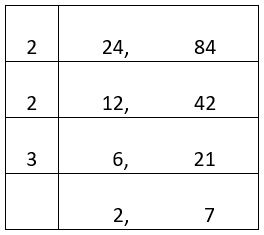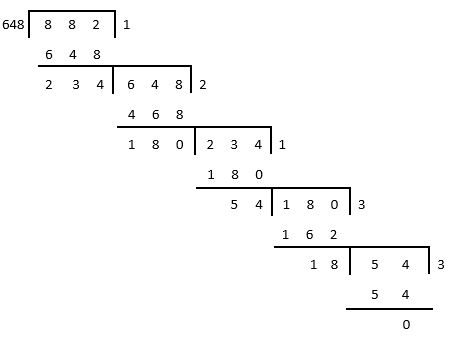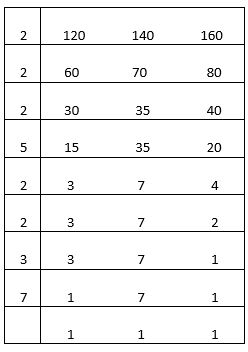## LetsPlayMaths.Com

WELCOME TO THE WORLD OF MATHEMATICS

# Class 6 Playing with Numbers

Factors and Multiples

Common Factors

Common Multiples

Even Number

Odd Number

Prime Number

Composite Number

Co-prime Number

Divisibility Rules of Natural Numbers

Divisible by 2

Divisible by 3

Divisible by 4

Divisible by 5

Divisible by 6

Divisible by 7

Divisible by 8

Divisible by 9

Divisible by 10

Divisible by 11

Prime Number check

Divisibility General Properties

HCF by Prime Factorization Method

HCF by Division Method

LCM by Division Method

Playing with Numbers Test

Playing with Numbers Worksheet

Answer Sheet

## Factors and Multiples

When two numbers are multiplied together then the result is called their product. Two numbers which are multiplied are known as Factor of the product and the product is known as Multiple of both the numbers. Let's see some examples.

Example 1. The product of 2 and 3 is 6. Here 2 and 3 are factor of 6 and 6 is multiple of 2 and 3.

Example 2. Find the factors of 12.

Solution. 1 x 12 = 12
2 x 6 = 12
3 x 4 = 12
So, the factors of 12 is 1, 2, 3, 4, 6 and 12.

Example 3. Find the factors of 18.

Solution. 1 x 18 = 18
2 x 9 = 18
3 x 6 = 18
So, the factors of 18 is 1, 2, 3, 6, 9 and 18.

Example 4. Find the factors of 24.

Solution. 1 x 24 = 24
2 x 12 = 24
3 x 8 = 24
4 x 6 = 24
So, the factors of 24

## Common Factors

Let's consider following examples.

Example 1. Find out the common factors of 12 and 18.

Solution. The factors of 12 are 1, 2, 3, 4, 6 and 12.
The factors of 18 are 1, 2, 3, 6, 9, and 18.
Observe that 1, 2, 3, and 6 are factors of both 12 and 18. They are known as common factors of both 12 and 18.

Example 2. Find the common factors of 6 and 15.

Solution. The factors of 6 are 1, 2, 3 and 6.
The factors of 15 are 1, 3, 5 and 15.
Observe that 1 and 3 are common factors of both 6 and 15.

## Common Multiples

Let's consider the following examples.

Example 1. Find out the common multiples of 2 and 3.

Solution. The multiples of 2 are 2, 4, 6, 8, 10, 12, 14, 16, 18, 20,...
The multiples of 3 are 3, 6, 9, 12, 15, 18, 21,...
Observe that 6, 12, 18, … are common multiples of both 2 and 3.

Example 2. Find out the common multiples of 4 and 6.

Solution. The multiples of 4 are 4, 8, 12, 16, 20, 24, 28, 32, 36, 40,...
The multiples of 6 are 6, 12, 18, 24, 30, 36, 42,...
Observe that 12, 24, 36,... are common multiples of 4 and 6.

## Even Number

A natural number which is exactly divisible by 2 is known as an Even Number. 2, 4, 6, 8, 10, 12, 14, 16,... are some even numbers.

## Odd Number

A natural number which is not exactly divisible by 2 is known as an Odd Number. 1, 3, 5, 7, 9, 11, 13, 15,... are some of the odd numbers.

## Prime Number

A natural number greater than 1 is known as a prime number if it's only factors are 1 and the number itself. 2, 3, 5, 7, 11, 13,... are some prime numbers.

## Composite Number

A Natural number is known as a composite number if it has more than two different factors. 4, 6, 8, 9, 10, 12, 14,... are some composite numbers.

## Co-prime Number

Two natural numbers are called Co-prime numbers if they have no common factor other than 1. Some examples are given below.

2, 3; 2, 5; 3, 5; 3, 4; 3, 8

## Divisibility Rules of Natural Numbers

Till now we know to test whether or not a number is divisible by another number is to perform actual division and see whether the remainder is zero. This is a time-consuming process, let's know some divisibility rules to know if a number is divisible by a certain number.

## Divisible by 2

A number is divisible by 2 if its last digit is divisible by 2. In other words, if the last digit of a number is 0, 2, 4, 6, 8 then the number is divisible by 2. Let's see some examples.

Examples 1. Check if 246 is divisible by 2.

Solution. Last digit of 246 is 6, and 6 is divisible by 2. So, 246 is divisible by 2.

Example 2. Check if 365 is divisible by 2.

Solution. Last digit of 365 is 5 and it is not divisible by 2. So, 365 is not divisible by 2.

## Divisible by 3

A number is divisible by 3 if the sum of it's digits is divisible by 3.

Examples 1. Check of 74526 is divisible by 3.

Solution. Sum of digits of 74526 = 7 + 4 + 5 + 2 + 6 = 24
24 is divisible by 3.
So, 74526 is divisible by 3.

Example 2. Check if 35407 is divisible by 3.

Solution. Sum of digits of 35407 = 3 + 5 + 4 + 7 = 19
19 is not divisible by 3.
So, 35407 is not divisible by 3.

## Divisible by 4

A number is divisible by 4 if the number formed by its last two digits is divisible by 4.

Example 1. Check if 54372 is divisible by 4.

Solution. Last two digits of 54372 is 72.
Since 72 is divisible by 4, the given number 54372 is divisible by 4.

Example 2. Check if 95365 is divisible by 4.

Solution. Last two digits of 95365 is 65.
Since 65 is not divisible by 4, the given number 95365 is not divisible by 4.

## Divisible by 5

A number is divisible by 5 if its last digit is 0 or 5.

Example 1. Check if 4560 is divisible by 5.

Solution. Last digit of 4560 is zero. So, 4560 is divisible by 5.

Example 2. Check if 9875 is divisible by 5.

Solution. Last digit of 9875 is 5. So, 9875 is divisible by 5.

Example 3. Check if 25872 is divisible by 5.

Solution. Last digit of 25872 is 2. So, it is not divisible by 5.

## Divisible by 6

A number is divisible by 6 if it is divisible by 2 and 3.

Example 1. Check if 5764 is divisible by 6.

Solution. Last digit of 5764 is 4 and it is divisible by 2. So, 5764 is divisible by 2.

Addition of all digits of 5764 = 5 + 7 + 6 + 4 = 22

22 is not divisible by 3. So, 5764 is not divisible by 3.

Hence 5764 is not divisible by 6.

Example 2. Check if 87234 is divisible by 6.

Solution. Last digit of 87234 is 4 and it is divisible by 2. So, 87234 is divisible by 2.

Addition of all digits of 87234 = 8 + 7 + 2 + 3 + 4 = 24

24 is divisible by 3. So, 87234 is divisible by 3.

Hence 87234 is divisible by 6.

## Divisible by 7

A number is divisible by 7 if the difference of sums of numbers in alternate blocks of three digits from right to left is divisible by 7.

Example 1. Check 34597436245 is divisible by 7.

Solution. The sum of numbers in alternate blocks of three digits from right to left are

245 + 597 = 842 and 436 + 34 = 470

Difference between 842 and 470 = 842 − 470 = 372

372 is not divisible by 7.

So, 34597436245 is not divisible by 7.

Example 2. Check if 126001169021 is divisible by 7.

Solution. The sum of numbers in alternate blocks of three digits from right to left are

021 + 001 = 22 and 169 + 126 = 295

Difference between 295 and 22 = 273

273 is divisible by 7.

So, 126001169021 is divisible by 7.

## Divisible by 8

A number is divisible by 8 if the number formed by its last three digits is divisible by 8.

Example 1. Check if 45096 is divisible by 8.

Solution. The number formed by the last three digits is 096 i.e. 96. We know 96 is divisible by 8.
Hence 45096 is divisible by 8.

Example 2. Check if 472124 is divisible by 8.

Solution. The number formed by the last three digits is 124 and it is not divisible by 8.
Hence 472124 is not divisible by 8.

## Divisible by 9

A number is divisible by 9 if the sum of its digits is divisible by 9.

Example 1. Check if 572349 is divisible by 9.

Solution. Sum of digits of 572349 = 5 + 7 + 2 + 3 + 4 + 9 = 30

30 is not divisible by 9.

Hence 572349 is not divisible by 9.

Example 2. Check if 435123 is divisible by 9.

Solution. Sum of digits of 435123 = 4 + 3 + 5 + 1 + 2 + 3 = 18

18 is divisible by 9.

Hence 435123 is divisible by 9.

## Divisible by 10

A number is divisible by 10 if its last digit is 0.

Example 1. Check if 2457890 is divisible by 10.

Solution. Last digit or unit place digit of 2457890 is 0. Hence 2457890 is divisible by 10.

Example 2. Check if 9784532 is divisible by 10.

Solution. Last digit or unit place digit of 9784532 is 2. Hence 9784532 is not divisible by 10.

## Divisible by 11

A number is divisible by 11 if the difference of the sum of digits at the odd place and the sum of digits at even place is either zero or divisible by 11.

Example 1. Check if 735243 is divisible by 11.

Solution. Sum of digits at odd place = 3 + 2 + 3 = 8

Sum of digits at the even place = 4 + 5 + 7 = 16

16 − 8 = 8

8 is not divisible by 11.

Hence 735243 is not divisible by 11.

Example 2. Check if 754633 is divisible by 11.

Solution. Sum of digits at odd place = 3 + 6 + 5 = 14

Sum of digits at the even place = 3 + 4 + 7 = 14

14 − 14 = 0

Hence 754633 is divisible by 11.

## Prime Number check

To decide whether a number is prime or not we need to follow the following steps. Let's assume the number is 'n'.

Step-1. Find the largest natural number 'm' such that m x m ⋜ n.

Step-2. Find all the prime numbers less than or equal to 'm'.

Step-3. If 'n' is not divisible by each prime number found in above step, then 'n' is a prime number.

Example 1. Check if 139 is a prime number.

Solution. 11 x 11 = 121      121 < 139

12 x 12 = 144      144 > 139

11 is the largest number such that 11 x 11 ⋜ 139.

Prime numbers less than or equal to 11 are 2, 3, 5, 7 and 11.

Note that 139 is divisible by 7.

Hence, 139 is not a prime number.

Example 2. Check if 229 is a prime number.

Solution. 15 x 15 = 225      225 < 229

16 x 16 = 256      256 > 229

15 is the largest number such that 15 x 15 ⋜ 229

Prime numbers less than or equal to 15 are 2, 3, 5, 7, 11 and 13.

Note that 229 is not divisible by 2, 3, 5, 7, 11 and 13.

Hence, 229 is a prime number.

## Divisibility General Properties

Overall, we have 4 divisible properties and they are provided below.

Property 1. If a number is divisible by another number, then it is divisible by each of the factors of that number.

Example 1. We know 54 is divisible by 18. The factors of 18 are 1, 2, 3, 6, 9 and 18. Now, we can confirm that 54 is divisible by 1, 2, 3, 6, 9 and 18.

Example 2. We know that 48 is divisible by 24. The factors of 24 are 1, 2, 4, 6, 8, 12, and 24. Now we can confirm that 48 is divisible by 1, 2, 4, 6, 8, 12 and 24.

Property 2. If a number is divisible by two co-prime numbers, then it is divisible by their product.

Example 1. 45 is divisible by both 3 and 5. Note that 3 and 5 are co-prime numbers and their product 15 is also factors of 45.

Example 2. 96 is divisible by both 3 and 8. Note that 3 and 8 are co-prime numbers and their product 24 is also factors of 96.

Property 3. If two numbers are divisible by a number, then their sum is also divisible by that number.

Example 1. 15 and 25 is divisible by 5. The sum of 15 and 25 (15 + 25 = 40) i.e. 40 is also divisible by 5.

Example 2. 24 and 48 is divisible by 8. The sum of 24 and 48 (24 + 48 = 72) i.e. 72 is also divisible by 8.

Property 4. If two given numbers are divisible by a number, then their difference is also divisible by that number.

Example 1. The number 50 and 40 both are divisible by 5. The difference of these numbers (50 − 40 = 10) i.e. 10 is also divisible by 5.

Example 2. The number 72 and 27 both are divisible by 9. The difference of these numbers (72 − 27 = 45) i.e. 45 is also divisible 9.

## HCF by Prime Factorization Method

In this method, we write the prime factorization of each of the given numbers. HCF is the product of all the common prime factors.

Example 1. Find out the HCF of 24 and 84.

Solution.HCF of 24 and 84 = 2 x 2 x 3 = 12

Example 2. Find out the HCF of 54, 72 and 90.

Solution.HCF of 54, 72 and 90 = 2 x 3 x 3 = 18

## HCF by Division Method

This method is more useful when the numbers are large. To find out the HCF of two numbers, we take the following steps given below.

a) First divide the larger number by smaller number.

b) Next, divide the previous divisor by the remainder in step A.

c) We then divide the new divisor of step B by the new remainder by the new remainder in step B.

d) Continue the process like step B till we get zero as remainder.

e) We write the last divisor as HCF of given numbers.

Example 1. Find the HCF of 882 and 648.

Solution.18 is the HCF of 648 and 882.

## LCM by Division Method

In this method, we take the numbers and divide them with the smallest possible prime factor. If any number gets divided fully then we divide it, else we keep it as it is. In the next step, we divide it by the next possible prime factor. We keep on doing it unless we get all the quotients become 1. In the end, we multiply all the prime factors to get the LCM.

Example 1. Find the LCM of 120, 140 and 160.

Solution.So, the LCM of 120, 140 and 160 = 2 X 2 X 2 X 5 X 2 X 2 X 3 X 7 = 3360

## Class-6 Playing with Numbers Test

Playing With Numbers Test - 1

Playing With Numbers Test - 2

## Class-6 Playing with Numbers Worksheet

Playing With Numbers Worksheet - 1

Playing With Numbers Worksheet - 2

Playing With Numbers Worksheet - 3

## Answer Sheet

Playing-with-Numbers-AnswerDownload the pdf

Copyright © 2021 LetsPlayMaths.com. All Rights Reserved.